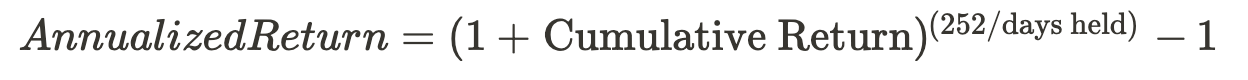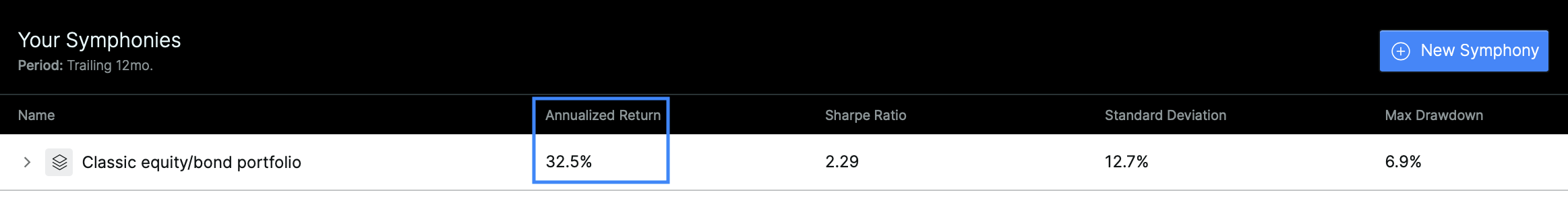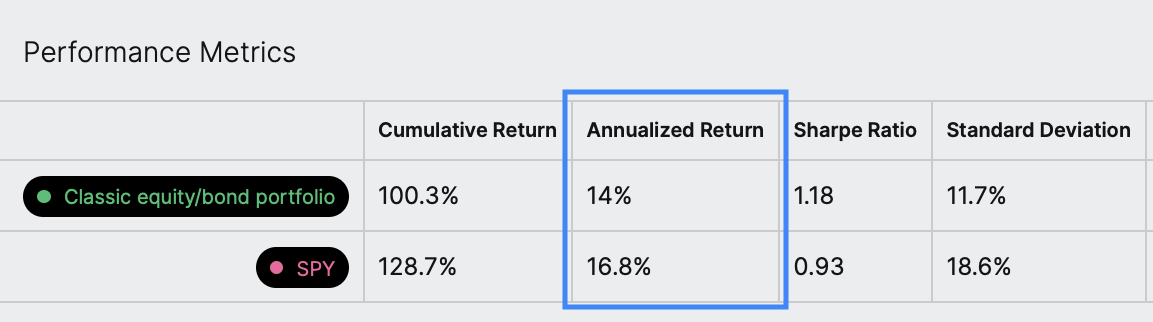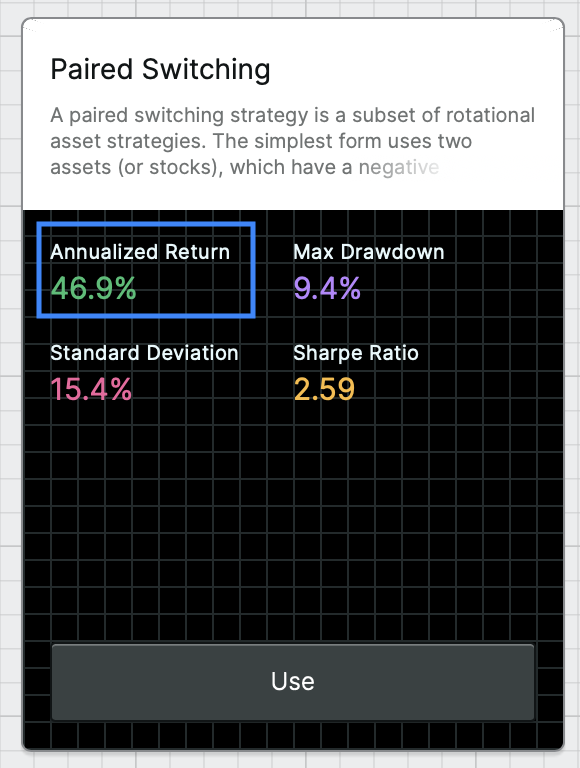# Annualized return

What is the annualized return?

The annualized rate of return is the geometric mean of the returns of an investment. It is a measure of how much that investment would earn on average per year, assuming the return was compounded, over a given time period.

What is the formula?Where will you see an annualized return in Composer?

This metric appears in a few places in Composer including:

• In your library of symphonies• In the results of a symphony backtest• In the overview of a symphony templateWhat is the step-by-step calculation?

Let's go through the calculation of the annualized return step-by-step for a symphony backtest. Here's how we do it:

• Compute the cumulative percent return of the symphony for the time between the first and last date in the backtest
• Add one to that value
• Raise that result to the (252/N) power, where N is the number of trading days in the backtest
• Subtract 1 from that result